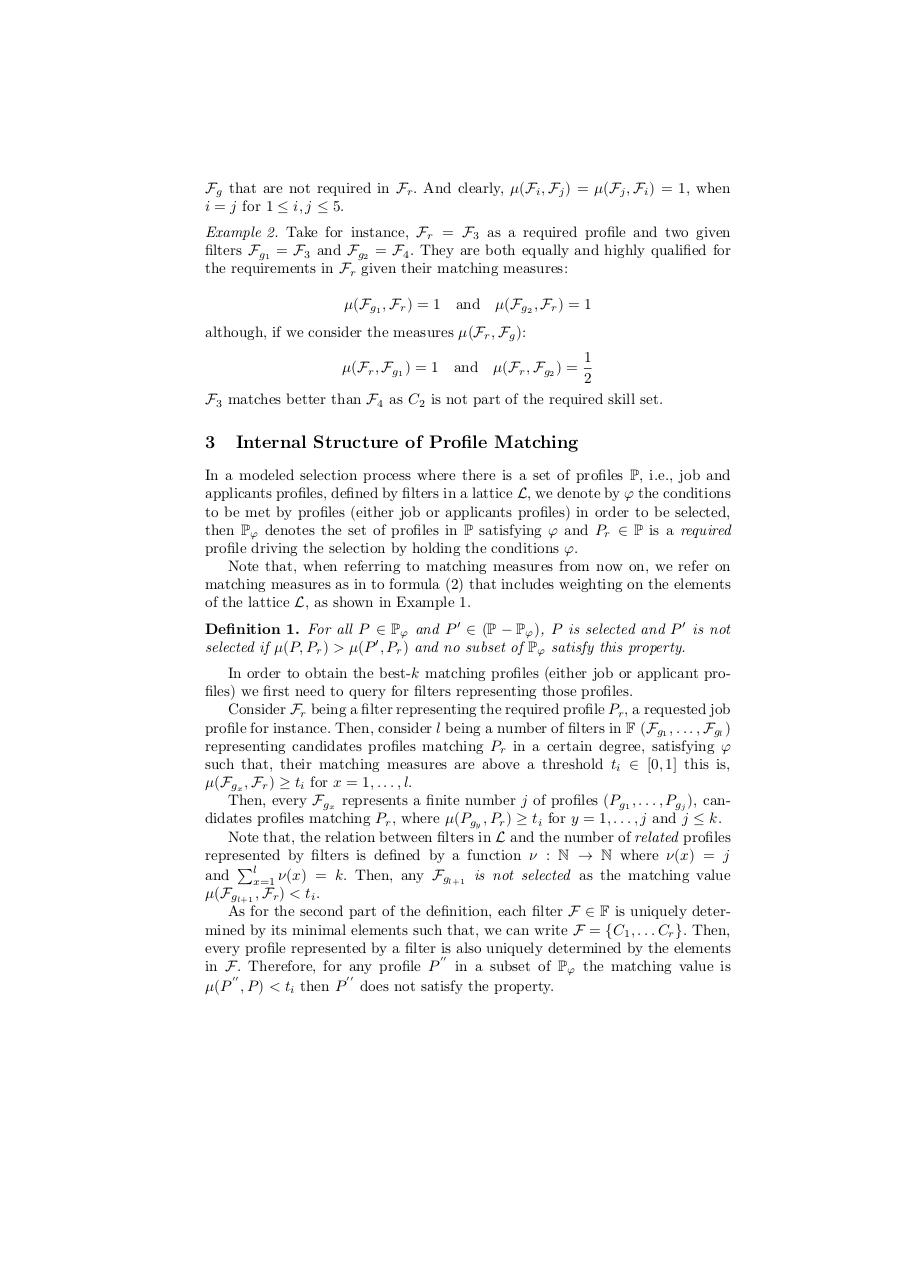# Top K Queries.pdfPage 1...3 4 56715

#### Text preview

Fg that are not required in Fr . And clearly, µ(Fi , Fj ) = µ(Fj , Fi ) = 1, when
i = j for 1 ≤ i, j ≤ 5.
Example 2. Take for instance, Fr = F3 as a required profile and two given
filters Fg1 = F3 and Fg2 = F4 . They are both equally and highly qualified for
the requirements in Fr given their matching measures:
µ(Fg1 , Fr ) = 1 and µ(Fg2 , Fr ) = 1
although, if we consider the measures µ(Fr , Fg ):
1
2
F3 matches better than F4 as C2 is not part of the required skill set.
µ(Fr , Fg1 ) = 1 and µ(Fr , Fg2 ) =

3

Internal Structure of Profile Matching

In a modeled selection process where there is a set of profiles P, i.e., job and
applicants profiles, defined by filters in a lattice L, we denote by ϕ the conditions
to be met by profiles (either job or applicants profiles) in order to be selected,
then Pϕ denotes the set of profiles in P satisfying ϕ and Pr ∈ P is a required
profile driving the selection by holding the conditions ϕ.
Note that, when referring to matching measures from now on, we refer on
matching measures as in to formula (2) that includes weighting on the elements
of the lattice L, as shown in Example 1.
Definition 1. For all P ∈ Pϕ and P ′ ∈ (P − Pϕ ), P is selected and P ′ is not
selected if µ(P, Pr ) &gt; µ(P ′ , Pr ) and no subset of Pϕ satisfy this property.
In order to obtain the best-k matching profiles (either job or applicant profiles) we first need to query for filters representing those profiles.
Consider Fr being a filter representing the required profile Pr , a requested job
profile for instance. Then, consider l being a number of filters in F (Fg1 , . . . , Fgl )
representing candidates profiles matching Pr in a certain degree, satisfying ϕ
such that, their matching measures are above a threshold ti ∈ [0, 1] this is,
µ(Fgx , Fr ) ≥ ti for x = 1, . . . , l.
Then, every Fgx represents a finite number j of profiles (Pg1 , . . . , Pgj ), candidates profiles matching Pr , where µ(Pgy , Pr ) ≥ ti for y = 1, . . . , j and j ≤ k.
Note that, the relation between filters in L and the number of related profiles
represented by filters is defined by a function ν : N → N where ν(x) = j
Pl
and
x=1 ν(x) = k. Then, any Fgl+1 is not selected as the matching value
µ(Fgl+1 , Fr ) &lt; ti .
As for the second part of the definition, each filter F ∈ F is uniquely determined by its minimal elements such that, we can write F = {C1 , . . . Cr }. Then,
every profile represented by a filter is also uniquely determined by the elements
′′
in F . Therefore, for any profile P in a subset of Pϕ the matching value is
′′
′′
µ(P , P ) &lt; ti then P does not satisfy the property.